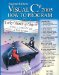# Passing Arrays and Array Elements to Methods

To pass an array argument to a method, specify the name of the array without any brackets. For example, if array hourlyTemperatures is declared as

```double[] hourlyTemperatures = new double[ 24 ];
```

then the method call

```ModifyArray( hourlyTemperatures );
```

passes the reference of array hourlyTemperatures to method ModifyArray. Every array object "knows" its own length (and makes it available via its Length property). Thus, when we pass an array object's reference to a method, we need not pass the array length as an additional argument.

For a method to receive an array reference through a method call, the method's parameter list must specify an array parameter. For example, the method header for method ModifyArray might be written as

```void ModifyArray( double[] b )
```

indicating that ModifyArray receives the reference of an array of doubles in parameter b. The method call passes array hourlyTemperature's reference, so when the called method uses the array variable b, it refers to the same array object as hourlyTemperatures in the calling method.

When an argument to a method is an entire array or an individual array element of a reference type, the called method receives a copy of the reference. However, when an argument to a method is an individual array element of a value type, the called method receives a copy of the element's value. To pass an individual array element to a method, use the indexed name of the array as an argument in the method call. If you want to pass a value-type array element to a method by reference, you must use the ref keyword as shown in Section 7.14, Passing Arguments: Pass-by-Value vs. Pass-by-Reference.

Figure 8.13 demonstrates the difference between passing an entire array and passing a value-type array element to a method. The foreach statement at lines 1718 outputs the five elements of array (an array of int values). Line 20 invokes method ModifyArray, passing array as an argument. Method ModifyArray (lines 3741) receives a copy of array's reference and uses the reference to multiply each of array's elements by 2. To prove that array's elements (in Main) were modified, the foreach statement at lines 2425 outputs the five elements of array again. As the output shows, method ModifyArray doubled the value of each element.

Figure 8.13. Passing arrays and individual array elements to methods.

(This item is displayed on pages 354 - 355 in the print version)

``` 1 // Fig. 8.13: PassArray.cs
2 // Passing arrays and individual array elements to methods.
3 using System;
4
5 public class PassArray
6 {
7 // Main creates array and calls ModifyArray and ModifyElement
8 public static void Main( string[] args )
9 {
10 int[] array = { 1, 2, 3, 4, 5 };
11
12 Console.WriteLine(
13 "Effects of passing reference to entire array:
" +
14 "The values of the original array are:" );
15
16 // output original array elements
17 foreach ( int value in array )
18 Console.Write( " {0}", value );
19
20 ModifyArray( array ); // pass array reference
21 Console.WriteLine( "

The values of the modified array are:" );
22
23 // output modified array elements
24 foreach ( int value in array )
25 Console.Write( " {0}", value );
26
27 Console.WriteLine(
28 "

Effects of passing array element value:
" +
29 "array before ModifyElement: {0}", array[ 3 ] );
30
31 ModifyElement( array[ 3 ] ); // attempt to modify array[ 3 ]
32 Console.WriteLine(
33 "array after ModifyElement: {0}", array[ 3 ] );
34 } // end Main
35
36 // multiply each element of an array by 2
37 public static void ModifyArray( int[] array2 )
38 {
39  for ( int counter = 0; counter < array2.Length; counter++ )
40  array2[ counter ] *= 2;
41 } // end method ModifyArray
42
43 // multiply argument by 2
44 public static void ModifyElement( int element )
45 {
46  element *= 2;
47  Console.WriteLine(
48  "Value of element in ModifyElement: {0}", element );
49 } // end method ModifyElement
50 } // end class PassArray
```

 ``` Effects of passing reference to entire array: The values of the original array are: 1 2 3 4 5 The values of the modified array are: 2 4 6 8 10 Effects of passing array element value: array before ModifyElement: 8 Value of element in ModifyElement: 16 array after ModifyElement: 8 ```

Figure 8.13 next demonstrates that when a copy of an individual value-type array element is passed to a method, modifying the copy in the called method does not affect the original value of that element in the calling method's array. To show the value of array[ 3 ] before invoking method ModifyElement, lines 2729 output the value of array[ 3 ] which is 8. Line 31 calls method ModifyElement and passes array[ 3 ] as an argument. Remember that array[ 3 ] is actually one int value (8) in array. Therefore, the application passes a copy of the value of array[ 3 ]. Method ModifyElement (lines 4449) multiplies the value received as an argument by 2, stores the result in its parameter element, then outputs the value of element (16). Since method parameters, like local variables, cease to exist when the method in which they are declared completes execution, the method parameter element is destroyed when method ModifyElement terminates. Thus, when the application returns control to Main, lines 3233 output the unmodified value of array[ 3 ] (i.e., 8).Visual C# 2005 How to Program (2nd Edition)
ISBN: 0131525239
EAN: 2147483647
Year: 2004
Pages: 600